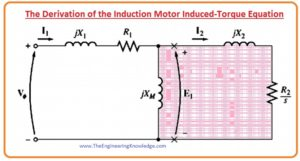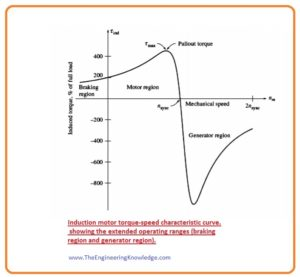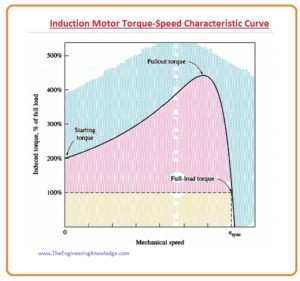Hello friends, I hope all of you are doing great. In today’s tutorial, we are gonna have a look at the Induction Motor Induced-Torque Equation. The torque induced in any induction motor can be elaborated as it is the torque produced when the electrical energy is converted into mechanical power.

The torque of the three-phase induction motor relies on the 3 very important parameters. The first one is the current induced in the rotor, 2nd is the stator flux that links with the rotor to produce voltage and 3rd is the P.F of the induction motor. In today’s post, we will relate all these three parameters with one another and find the induction motor torque equation by using the torque-speed characteristics and equivalent circuit of the induction motor. So let’s get started with Derivation of the Induction Motor Induced-Torque Equation.

#### Derivation of the Induction Motor Induced-Torque Equation

• It is likely to usage the correspondent circuitry of an induction motor and the power movement (flow) figure for the motor to find a universal expression for induced torque as a function of speed.
• The induced torque (tind) in the motor can be found by these two expressions.

Tind = Pconv /wm

Tind = PAG/Wsync

• The second equation is particularly valuable, as the synchronous speed (Wsync) is a persistent (constant) for a given frequency (f) and the number of poles (p).
• As the (Sync) has constant value so we can find the induced torque (tind) of the motor by the air-gap power (PAG).
• The air-gap power (PAG) is the power crossing the (gap) from the stator circuitry to the rotor circuitry. It is equivalent to the power consumed in the resistor R2/s.
• Mention to the equivalent circuit of induction motor which is shown in the given diagram.• In this picture, the air-gap power (PAG)  provided to the single-ø of the motor can be given by this equation.

(PAG) 1ø = I22 (R2/s)

• So, the power gap for three-phase or total will be.

(PAG) = 3I22 (R2/s)

• If we find the value of I2 then we can easily find the value power air gap (PAG) and induced torque (tind).

#### Thevenin Theorem to find the Induced Torque of Induction Motor

``````
• To compute the Thevenin corresponding of the input part of the induction motor correspondent circuitry, first open-circuitry the points at the (X’s) and calculate the resultant open-circuitry voltage (V) existing there.
• To measure the Thevenin impedance (Z), short-circuit the phase voltage (V) and calculate the (Zeq) seeing into the terminals.
• In the given diagram (A) label circuit shows the open terminals used to calculate the Thevenin voltage (VTH). By using the voltage divider rule we get.

(VTH)= Vø(ZM /ZM +Z1)

= Vø(jXM /R1+JX1+jXM)

• The magnitude of the Thevenin voltage (VTH) is given as.

(VTH)= Vø(XM / √(R21+(X1+XM)2)

• As the magnetization reactance XM >> X1 and XM >> R1, the magnitude of the Thevenin voltage (VTH) is about.

VTH = Vø(XM /XM +X1)

• The circuit which is labeled as (B) diagram shows that input circuitry with the input voltage source short circuit. The impedance (Z) in parallel assembly and the Thevenin impedance (ZTH)is denoted as.

ZTH = (Z1ZM)/ (Z1+ZM )

ZTH = RTH + JXTH = jXM(R1+jX1)/(R1+j(X1+ XM))

• As the XM >> X1 and XM + X1 >> R1 the Thevenin resistance(RTH) and reactance (XTH) are about given as.

RTH=R1 (XM/X1+ XM )2

XTH = X1

• The subsequent corresponding circuitry is labeled in diagram as (C).
• From this circuitry, the current (12) is given as.

I2= (VTH/ZTH+Z2)

(VTH/ZTH+R2/s+jXTH+jX2)

• The magnitude of this current(I2) is given as.

I2 = VTH/√(RTH+R2/s)2+ (XTH+X2)2

• The air-gap power (PAG) is therefore given by.

PAG = 3I22(R2/s)

= (3VTHR2/s)/[(RTH+R2/s)2+(XTH+X2)2]

• The induced torque (tind) is given as.

Tind = PAG/Wsync

Tind= (3VTHR2/s)/ Wsync[(RTH+R2/s)2+(XTH+X2)2]

#### Comments on the Induction Motor Torque-Speed Curve

• There are two diagrams given below the first one is the induction motor torque-speed characteristic curve while other diagram offers numerous significant info about the process of induction motors. Now we discuss this information.• The induced torque (Tind) of the motor is 0 at synchronous speed (Wsync).
• The torque-speed graph is almost rectilinear (linear) among zero load and full load conditions. In this assortment, the rotor resistor (RR) is larger than the reactance of rotor (XR), so the rotor current (IR), the rotor field (BR), and the induced torque (Tind) upsurge linearly with increment in the slip.
• There is an extremely probable torque that cannot be surpassed. This torque (t), is known as the pullout torque which is two to three times the rated full-load torque of the motor. We also have discussed the pullout torque of the induction motor.
• The initial torque on the motor is somewhat greater than its pullout torque (full-load torque), thus the motor will start taking any load which it can supply at full-power.
• Note that the torque on the motor for a specified slip (S) fluctuates as the square of the provided voltage (V).
• If the rotor of the induction motor is moving faster than synchronous speed (Wsync), then the movement of the induced torque (Tind) in the motor converses and the motor behaves like a generator, changing mechanical power to electrical power.• If the motor is rotating regressive comparative to the path of the magnetic fields, the induced torque (tind) will halt the motor very quickly and will attempt to move it on the other track.
• Meanwhile changing the way of the magnetic field (B) revolution is merely a substance of swapping any 2 stator phases, this detail can be used as a method to very quickly halt an induction motor. The changing of the 2 phases for stopping the motor very quickly is named as plugging.
• The power converted (Pconv) to mechanical in an induction motor is given as.

Pconv = Tind x Wsync

• It shown in the given diagram, note that the highest power provided by the induction motor exits at a dissimilar speed than the maximum torque (Tmax) and, of course, no power is transformed to mechanical power when the speed of the rotor is 0.It is the complete and detailed article on the Induction Motor Induced-Torque Equation if you have any queries ask in comments. See you in the next tutorial, Maximum (Pullout) Torque in an Induction Motor.

You can also read some related articles to the induction motor. That is described here.

# Free SMT Assembly Monthly

New arrival for Aluminum boards, only \$2